# Engineering Mechanics - Force System Resultants

### Exercise :: Force System Resultants - General Questions

• Force System Resultants - General Questions
6.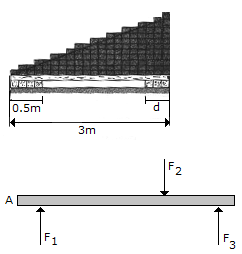The bricks on top of the beam and the supports at the bottom create the distributed loading shown in the second figure. Determine the required intensity w and dimension d of the right support so that the resultant force and couple moment about point A of the system are both zero.

 A. w = 175.0 N/m, d = 1.5 m B. w = 138.2 N/m, d = 1.9 m C. w = 125.0 N/m, d = 2.1 m D. w = 154.4 N/m, d = 1.7 m

Explanation:

No answer description available for this question. Let us discuss.

7.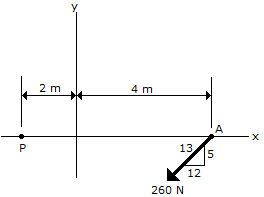Determine the magnitude and direction of the moment of the force of the movement if the force at A about point P.

 A. Mp = 1440 Nm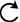B. Mp = 600 Nm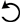C. Mp = 1440 NmD. Mp = 600 NmExplanation:

No answer description available for this question. Let us discuss.

8.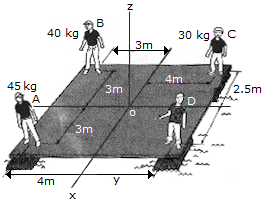The boys A, B and C stand near the edges of a raft as shown. Determine the location (x, y) of boy D so that all four boys create a single resultant force acting through the raft's center O. Provided the raft itself is symmetric, this would keep the raft afloat in a horizontal plane. the mass of each boy is indicated in the diagram.

 A. x = 4.5 m, y = 1.5 m B. x = 3.0 m, y = 3.0 m C. x = 1.5 m, y = 4.5 m D. x = 3.0 m, y = 4.0 m

Explanation:

No answer description available for this question. Let us discuss.

9.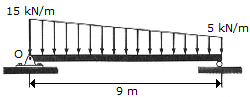Replace the loading by an equivalent force and couple moment acting at point O.

 A. R = 90 kN, M = 473 kN-m CW B. R = 90 kN, M = 338 kN-m CW C. R = 45 kN, M = 203 kN-m CW D. R = 135 kN, M = 270 kN-m CW

Explanation:

No answer description available for this question. Let us discuss.

10.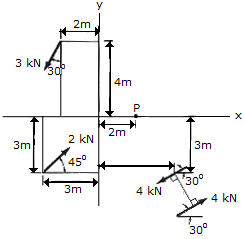Replace the force and couple system by an equivalent single force and couple acting at point P.

 A. F = (-0.0858i-1.184j) kN, M = 36.6 kN-mB. F = (-0.0858i-1.184j) kN, M = 21.6 kN-mC. F = (-0.0858i-1.184j) kN, M = 13.61 kN-mD. F = (-0.0858i-1.184j) kN, M = 35.7 kN-m